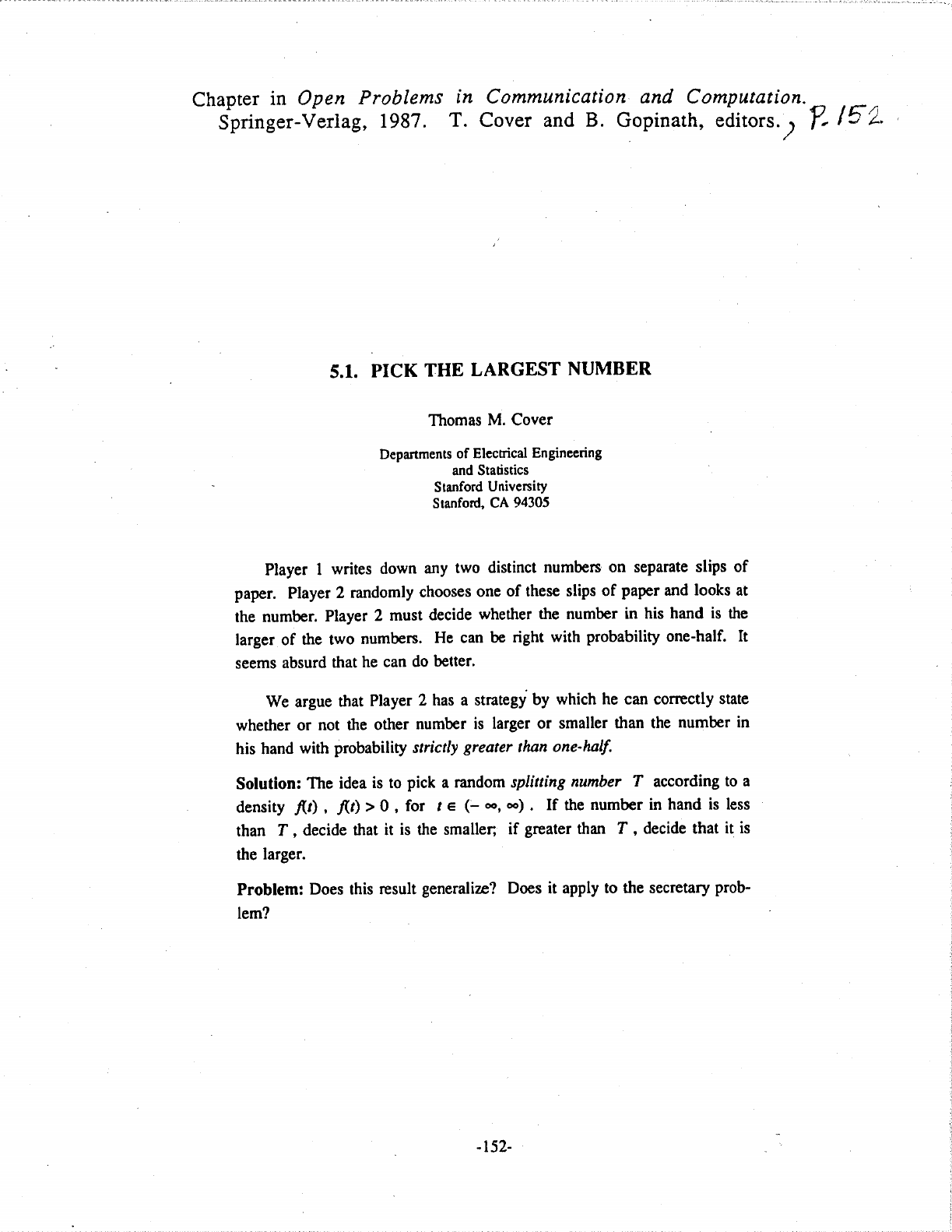Thomas Cover was an information theorist and professor at Stanford ...
When you pick a random number T, there are 3 possible scenarios. ...
This solution might be a little hard to grasp because regardless of...Thomas Cover was an information theorist and professor at Stanford University. The game he talks in the paper is a specific case of a 1958 betting game called ‘Googol’. In 'Googol' you ask someone to take as many slips of paper as he pleases, and on each slip write a different positive number. The numbers may range from small fractions of 1 to a number the size of a googol (1 followed by a hundred 0s) or even larger. These slips are turned face down and shuffled over the top of a table. One at a time you turn the slips face up. The aim is to stop turning when you come to the number that you guess to be the largest of the series. You cannot go back and pick a previously turned slip. If you turn over all the slips, then of course you must pick the last one turned. Martin Gardner mentioned it in his February 1960 column in Scientific American, but he disregarded the case for just 2 slips of paper considering it to be trivial. This is exactly the case that the author investigates in this paper. ![](https://news.stanford.edu/news/gifsarch2/cover250-412.jpg) This solution might be a little hard to grasp because regardless of your choice of T there will always be infinite numbers above and below it. An alternative and maybe more intuitive way to attack this problem is to first map the real line into the open interval (0,1). Note that the cardinality (number of elements) of these 2 sets is the same but we are not dealing with infinites any more. A way to do it is to use a function like $$f(x) = \frac{e^x}{e^x+1}$$ which maps $\mathbb{R} \rightarrow (0,1)$ and is strictly monotonic. ![](https://i.imgur.com/83ycbmv.png) Now, after picking the number from one of the slips of paper (A), you calculate f(A) and compare it with a random number T from the interval (0,1). If T < f(A), then A is the greater number, if T > f(A), then A is the smaller number. With this strategy, the probability of guessing the larger number is $$\frac{1}{2}f(Larger \ Number)+ \frac{1}{2}(1-f(Smaller \ Number))$$ where $\frac{1}{2}f(Larger \ Number)$ is the probability of choosing the larger number $1/2$ and then sticking with it $f(Larger \ Number)$ and $\frac{1}{2}(1-f(Smaller \ Number))$ is the probability of choosing the smaller number $1/2$ and then switching $1-f(Smaller \ Number)$. Computing the probabilities $$\frac{1}{2}f(Larger \ Number)+ \frac{1}{2}(1-f(Smaller \ Number)) = \\ \frac{1}{2}+\frac{1}{2}(f(Larger \ Number)-f(Smaller \ Number))$$ But $f(Larger \ Number)>f(Smaller \ Number)$ since f is strictly monotonic and so the probability of finding the larger number is always > 1/2! When you pick a random number T, there are 3 possible scenarios. Scenario 1: T is less than A and B ![](https://i.imgur.com/p3YWkD2.png) In this case, the chance of picking the larger number B is 50% Scenario 2: T is greater than A and B ![](https://i.imgur.com/IdUELQN.png) In this case, the chance of picking the smaller number A is also 50%. Scenario 3: T is in between A and B ![](https://i.imgur.com/UCtZfTn.png) Finally in this scenario, when you see A you should switch and pick the other envelope, whereas if you see B you should stay with B. The probability of finding the larger number is always 100%! The possibility of getting the largest number when you take into account the 3 scenarios is > 50%, even if just by a tiny amount. It's also interesting to note that this is true regardless of the number we end up picking, since there's always a non-zero probability that there are numbers above and below T.